### Computational Method

Let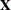represent the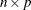design matrix and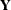the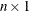vector of dependent variables. (See the section Parameterization of PROC GLM Models for information about howis formed from your model specification.)

The normal equations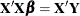are solved using a modified sweep routine that produces a generalized inverse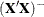and a solution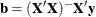. The modification is that rows and columns corresponding to diagonal elements that are found during sweeping to be zero (or within the expected level of numerical error of zero) are zeroed out. The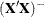produced by this procedure satisfies the following two equations: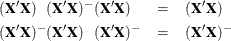Pringle and Rayner (1971) call a generalized inverse with these characteristics a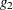-inverse, and this is the term usually used in SAS documentation and output. Urquhart (1968) uses the term reflexive g-inverse to emphasize thatis a generalized inverse ofin the same way thatis a generalized inverse of. Note that a-inverse is not necessarily unique: ifis singular, then sweeping the matrix in a different order will result in a different-inverse that also satisfies the two preceding equations.

For each effect in the model, a matrixis computed such that the rows ofare estimable. Tests of the hypothesis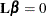are then made by first computing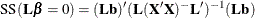and then computing the associated F value by using the mean squared error.